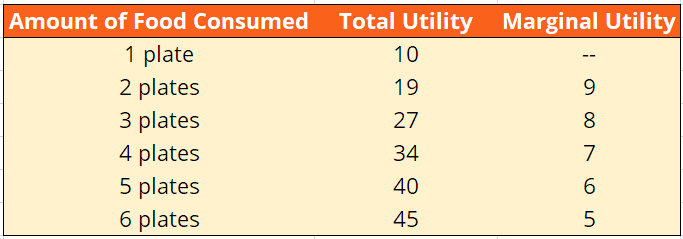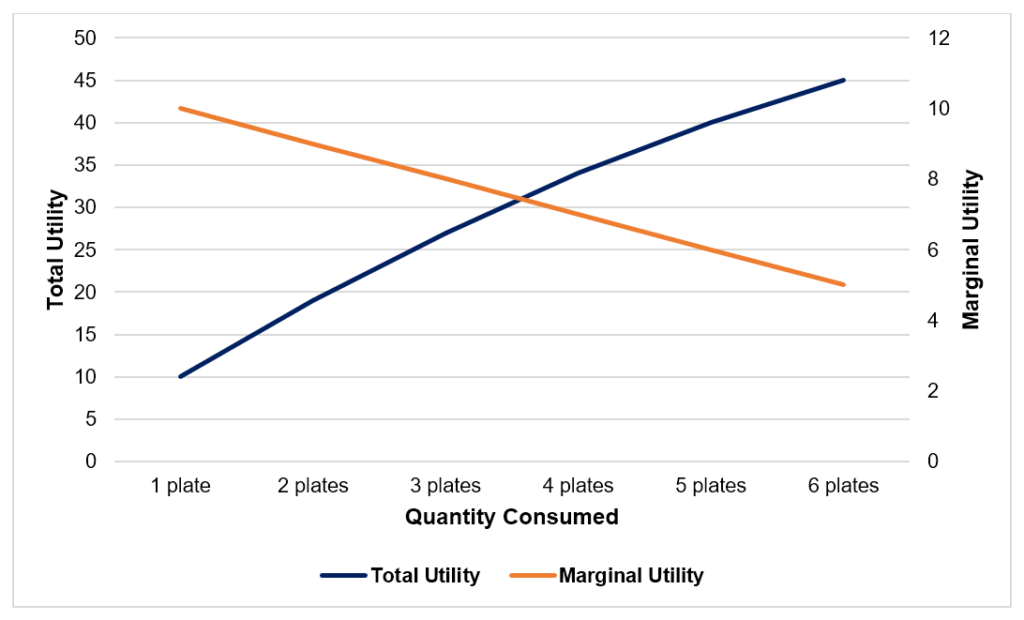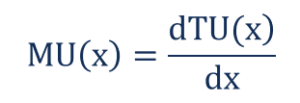# Law of Diminishing Marginal Utility

The additional utility gained from an increase in consumption decreases with an increase in the level of consumption

## What is the Law of Diminishing Marginal Utility?

The Law of Diminishing Marginal Utility states that the additional utility gained from an increase in consumption decreases with each subsequent increase in the level of consumption. Marginal Utility is the change in total utility due to a one-unit change in the level of consumption. The Law of Diminishing Marginal Utility states the marginal utility gradually decreases with the level of consumption.### Total Utility vs. Marginal Utility

Total Utility is an aggregate measure of satisfaction gained from consumption whereas Marginal Utility is a measure of the change in satisfaction gained from consumption as a result of a change in consumption.

#### MU(x) = TU(x) – TU(x – 1)

The Marginal Utility gained from the xth unit of consumption is equal to the difference between the total utility gained from x units of consumption and the total utility gained from x–1 units of consumption.

### The Law of Diminishing Marginal Utility – An Analogy

The Law of Diminishing Marginal Utility is best understood through an analogy. Consider the following example:

John is extremely hungry and goes to a restaurant that offers a buffet. He loads up his plate with food and starts eating. The amount of satisfaction gained by John from a plate of food is directly proportional to John’s hunger level. Therefore, the first plate of food will give John more satisfaction (utility) than the second plate of food, which in turn will give John more satisfaction than the third plate of food.

The situation above occurs because each plate of food reduces John’s hunger level. The reduction in hunger level results in less satisfaction from the plate of food being consumed. Each plate of food fills John up and thus lessens the amount of satisfaction he will get from the plates of food that follow. Mathematically, it can be represented by the following table:### Graphical Representation of the Law of Diminishing Marginal Utility### Mathematical Interpretation of the Law of Diminishing Marginal Utility

The law of diminishing marginal utility states the utility function is upward sloping and concave. Neoclassical microeconomic theory assumes that all commodities are infinitely divisible. This allows economists and mathematicians to assume continuous utility functions and use calculus to analyze marginal changes.

The example above implicitly makes use of the assumption of continuity. For instance, one can read off the graph that 3.5 plates of food give the consumer 27.5 units of utility. If we assume a continuous utility function, then the marginal utility from the xth unit of consumption is simply the slope (or derivative) of the total utility function at x units.### More Resources

CFI is the official provider of the Financial Modeling and Valuation Analyst (FMVA)™ certification program, designed to transform anyone into a world-class financial analyst.

To keep learning and developing your knowledge of financial analysis, we highly recommend the additional CFI resources below:

• Economic Rent
• Price Elasticity
• Supply and Demand
• Utility Theory

### Financial Analyst Certification

Become a certified Financial Modeling and Valuation Analyst (FMVA)® by completing CFI’s online financial modeling classes!